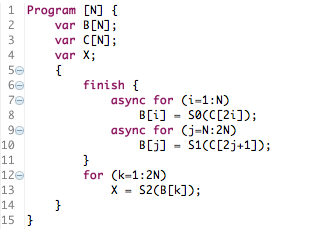Polyhedral X10 Dataflow Analyzer

Polyhedral X10 Dataflow Analyzer is a prototype implementation of an extension to Array Data-flow analysis for a subset of X10 programs. Our proposed technique will appear in PPoPP 2013. We restrict the programs to be affine control, and support a subset of parallel constructs; "async" and "finish". For this class of X10 programs, our analysis provides instance-wise and element-wise dependence information.

We apply the dependence information for detecting data races in X10 programs. The data-flow analysis answers the question: which instance of which statement produced the value read by an instance of a statement. For parallel programs with data races, a single read has multiple writers. This is the basic idea behind applying data-flow analysis to data-race detection.

We use a simplified mini-X10 language that strips out unnecessary information for our analysis. For example, since the operations performed in a statement are not relevant to dependence analysis, and so the right hand side expressions in all assignement statements are simply labeled functions. The mini-language looks like the following.Note how the RHS of assignment statements are just functions that take as parameters, the values of instances of array variables in the program. Since the details of the operations are not relevant, all statements are expressed as such functions (the function names mut all be unique). In the above example, instances of statement S0 and S1 execute in parallel. These two statements do not conflict for most iterations, but B[N] is written by both the statements, causing data-race.

In response to a series of "Questions," asking for the source(s) of a specific read reference, our analysis produces the following output:

Question (S0, C[2*i]):
input dependence

Question (S1, C[2*j + 1]):
input dependence

Question (S2, B[k]):
S0[k]
IF {k|k-1>=0 && -k+2N>=0 && -k+N>=0}

S1[k]
IF {k|k-1>=0 && -k+2N>=0 && k-N>=0}
and additionally, reports any data-races detected as ambiguous sources:

[Ambiguous Source] S2(B[k]) has two sources S0[k] and S1[k] at [N] -> {[k] : (k + -1*N = 0) and (-1 + N >= 0)}

The output lists the data-flow analysis questions, and the corresponding answer in the form of a Quast (quasi-affine solution tree, that can be viewed as a piecewise affine function). In the above, Question (S2, B[k]) asks which instance of which statement produced values read by reference B[k] in statement S2. The answer to the question is a quast with two branches:

• Producer is S0[k] (k-th iteration of statement S0) when 1≤ k ≤ N
• Producer is S1[k] (k-th iteration of statement S1) when N≤ k ≤ 2N
and the ambiguous write for k=N is reported separately.

Please refer to the documentation in our distribution for further information regarding the syntax of input language and outputs.

System Requirements:
• Java Runtime Environment 1.6 or higher.
• Mac or Linux. Windows OS is not supported.

Our system uses a number of tools developed for Eclipse, such as the Xtext parser generator, and requires some of the libraries from Eclipse. In addition, we have an editor integrated with Eclipse that provides syntax highlighting and context assists (which come for free when using Xtext).

We provide an Eclipse bundle for a version of our tool integrated into Eclipse.

• Eclipse Bundle for MacOSX (64bit)
• Eclipse Bundle for Linux (32bit)
• Eclipse Bundle for Linux (64bit)
• Test Project for Eclipse
(Start import wizard from Import... -> General -> Existing Projects into Workspace, and then select from archive)

We also provide a standalone, command line, version of our tool. Invoking the standalone version looks like the following (the questions are in comments at the end of the source and the system prints out modified comments as the answers):

\$java -jar polyX10.jar x10src/examplePaper2.x10p
Program [N] {
var B[N]
var C[N]
var X

{
finish {
async for (i=1:N)
B[i] = S0(C[2i]);

async for (j=N:2N)
B[j] = S1(C[2j+1]);
}

for (k=1:2N)
X = S2(B[k]);
}
}

/*
[Ambiguous Source] S2(B[k]) has two sources S0[k] and S1[k] at [N] -& {[k] : (k + -1*N = 0) and (-1 + N &= 0)}
Question (S2, B[k]):
S0[k]
IF {k|k-1&=0 && -k+2N&=0 && -k+N&=0}
S1[k]
IF {k|k-1&=0 && -k+2N&=0 && k-N&=0}

*/